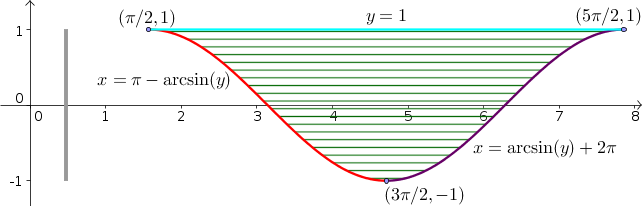# Math Insight

### Image: Change order of integration example region with sinusoid, x firstExample region illustrating process of changing the order of integration in double integrals, shown with $x$ as the inner integral. For a given value of $y$, $x$ ranges from the curve $x=\pi - \arcsin y$ (red) to the curve $x=2\pi +\arcsin y$ (purple), as illustrated by the horizontal hashing over the region. The total range of $y$ over the region is from $-1$ to 1, as illustrated by the gray bar at the left.

Source image file: double_integral_change_order_example_sin_x.ggb
Source image type: Geogebra